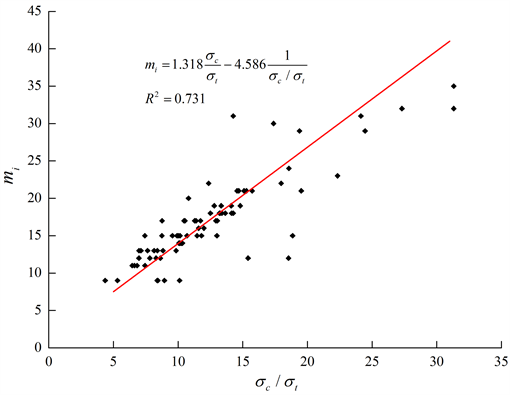# 一种岩石I型断裂韧度KIc估算方法An Estimation Method for Mode I Fracture Toughness KIc of Rocks

DOI: 10.12677/HJCE.2018.71010, PDF, HTML, XML, 下载: 1,014  浏览: 4,654

Abstract: The mode I fracture toughness KIc of rocks is a mechanical properties index which characterizes the resistance of instability expansion of inner cracks, namely brittle fracture damages of materials, but it is usually difficult to obtain this parameter in laboratory test. Therefore, based on the equivalent principle of uniaxial tensile strength of rocks, combining the relationship between mode I fracture toughness KIc and tensile strength of rocks with the relationship between experience parameter mi of Hoek-Brown (H-B) strength criterion and uniaxial compressive, tensile strength of rocks, a new estimation method of mode I fracture toughness KIc of rocks based on uniaxial compressive strength and experience parameter mi of rocks is proposed. Lastly, the reliability of the correlation is validated by some related testing results. When the basic testing data are unavailable, the proposed correlation can provide a preliminary estimation value of mode I fracture toughness KIc by the uniaxial compressive strength of rocks.

1. 引言

2. 岩石I型断裂韧度KIc与抗拉强度的关系分析

${K}_{\text{I}c}={\sigma }_{t}\sqrt{2\pi r}$ (1)

3. 岩石参数mi与抗拉、抗压强度的关系分析

H-B强度准则中反映不同类型岩石软硬程度的经验参数mi提供的只是一个范围值，而更加精确的mi取值尚需通过一系列的室内岩石力学试验获得，如根据岩石三轴压缩试验数据进行拟合。因此，研究者从理论或数据拟合角度已进行了大量的参数mi的试验取值研究，并建立参数mi与岩石间接拉伸强度σtB或直接拉伸强度σt、单轴抗压强度σc等的经验关系式，表2列出用数据统计方法给出的一些经验公式。

Cai由Griffith强度理论推得脆性材料的抗拉强度为抗压强度的1/8  ，岩石单轴抗拉强度与抗压强度的比值大致分布在区间1/10~1/20，该结论已为较多研究者提出  ，由本文统计的岩石强度参数计算Table 1. Empirical formulas of mode I fracture toughness and tensile strength of rocksTable 2. The experience relations between mi and strength parameters of rocksTable 3. Part of statistical results of uniaxial compressive strength and tensile strength of rocks

${m}_{i}=a\frac{{\sigma }_{c}}{{\sigma }_{t}}-b\frac{{\sigma }_{t}}{{\sigma }_{c}}$ (2)

${m}_{i}=1.318\frac{{\sigma }_{c}}{{\sigma }_{t}}-4.586\frac{{\sigma }_{t}}{{\sigma }_{c}}=1.318\frac{{\sigma }_{c}}{{\sigma }_{t}}-4.586\frac{1}{{\sigma }_{c}/{\sigma }_{t}}$ , R2 = 0.731 (3)

1) 岩石参数mi是基于三轴试验并且围压通常很大而获得的一经验值，部分岩石的取值区间较大，最大差值可达10，对于单轴拉伸或压缩应力状态下可能不太适用，针对某一特定岩石合理而准确地确定其mi值存在较大的困难。

2) 鉴于岩石强度测试为破坏性试验以及试件数量的多寡等原因，故在部分统计结果中，岩石的单轴抗压强度与其抗拉强度可能不具有代表性。Figure 1. The relationship between parameter mi and ratio of σct

4. 岩石I型断裂韧度KIc确定新方法及验算

4.1. 岩石I型断裂韧度KIc确定新方法

${K}_{Ιc}=-\frac{{\sigma }_{c}\left({m}_{i}-\sqrt{{m}_{i}{}^{2}+24.177}\right)}{63.103}$ (4)Table 4. Calculation results by the modified probational correlation

4.2. 岩石I型断裂韧度KIc确定新方法的验算

5. 结论与建议

1) 岩石参数mi与抗拉、抗压强度之间的经验关系式可表示为mi = 1.318σct − 4.856σtc，R2 = 0.731。由此关系式，即可由岩石参数mi和单轴抗压强度得到抗拉强度预测值。

2) 利用关系式 ${K}_{Ιc}=-\frac{{\sigma }_{c}\left({m}_{i}-\sqrt{{m}_{i}{}^{2}+24.177}\right)}{63.103}$ ，即可通过岩石单轴抗压强度并结合H-B强度准则中

3) 岩石经验参数mi与其抗拉、抗压强度之间的相关关系可能因岩石类型不同而相差较大。以上建议的三者之间的经验关系式，其相关系数R2并不高，通过岩石单轴抗压强度估算的部分I型断裂韧度KIc与实测值相差较大。针对不同岩石类型建立参数mi以及断裂韧度KIc与其强度参数之间的经验关系有待于进一步的理论和试验研究。

  朱传云. 岩石抗压强度与断裂韧度规律的探讨[J]. 水利水电技术, 1989(4): 5-6.  陈建国, 邓金根, 袁俊亮, 等. 页岩储层I、II型断裂韧性评价方法研究[J]. 岩石力学与工程学报, 2015, 34(6): 3.  陈治喜, 陈勉, 金衍, 等. 水压致裂法测定岩石的断裂韧性[J]. 岩石力学与工程学报, 1997, 16(1): 59-64.  侍克斌, 李新, 詹新武, 等. 运用断裂力学分析碾压混凝土坝表面裂缝[J]. 人民长江, 2008, 39(8): 48-51.  姜傅之. 断裂力学与裂纹扩展[M]. 北京: 北京航空学院出版社, 1984.  Whittaker, B.N., Singh, R.N. and Sun, G. (1992) Rock Fracture Mechanics Principles, Design and Applications. Elsevier, Amsterdam.  傅钢, 李贺, 鲜学福, 等. 压剪应力状态下脆性岩石复合断裂准则的研究[J]. 重庆大学学报自然科学版, 1990(2): 73-78.  李世愚. 岩石断裂力学导论[M]. 合肥: 中国科学技术大学出版社, 2010.  潘别桐, 唐辉明. 岩石压剪性断裂特性及I-II型复合断裂判据[J]. 地球科学, 1988(4): 59-67.  邓华锋, 朱敏, 李建林,等. 砂岩I型断裂韧度及其与强度参数的相关性研究[J]. 岩土力学, 2012, 33(12): 3585-3591.  臧启山, 姚弋. 工程断裂力学简明教程[M]. 合肥: 中国科学技术大学出版社, 2014.  Colak, K. and Unlu, T. (2004) Effect of Transverse Anisotropy on the Hoek-Brown Strength Parameter ‘mi’ for Intact Rocks. International Journal of Rock Mechanics & Mining Sciences, 41, 1045-1052. https://doi.org/10.1016/j.ijrmms.2004.04.004  朱合华, 张琦, 张连洋. Hoek-Brown强度准则研究进展与应用综述[J]. 岩土力学与工程学报, 2013, 32(10): 1945-1963.  Zhang, Z.X. (2002) An Empirical Relation between Mode I Fracture Tough-ness and the Tensile Strength of Rock. International Journal of Rock Mechanics & Mining Sciences, 39, 401-406. https://doi.org/10.1016/S1365-1609(02)00032-1  Richards, L.R. and Read, S.A.L. (2011) A Comparison of Methods for Determining mi, the Hoek-Brown Parameter for Intact Rock Material. 45th US Rock Mechanics/Geomechanics Symposium, San Francisco, 26-29 June 2011, ARMA-11-246.  Gercek, H. (2002) Properties of Failure Envelopes and Surfaces Defined by the Hoek-Brown Failure Criterion. Proceedings of the 6th Regional Rock Mechanics Symposium, Konya, 10-11 October 2002, 3-11.  Cai, M. (2010) Practical Estimates of Tensile Strength and Hoek-Brown Strength Parameter mi of Brittle Rocks. Rock Mechanics and Rock Engineering, 43, 167-184. https://doi.org/10.1007/s00603-009-0053-1  陈招军, 王乐华, 金晶, 等. 三种岩石巴西劈裂及单轴压缩试验对比研究[J]. 水力发电, 2015, 41(11): 34-38.  蒋伟. 不同岩石抗拉与抗压实验对比研究[D]: [硕士学位论文]. 南京: 南京大学, 2014.  王羽, 许强, 柴贺军, 等. 工程岩爆灾害判别的RBF-AR耦合模型[J]. 吉林大学学报(地), 2013, 43(6): 1943-1949.  孙丽. 论述岩石抗拉强度与单轴抗压强度两者之间的联系[J]. 农业科技与信息, 2012(14): 48-49.  Zhang, L. (2005) Engineering Properties of Rocks. Elsevier, New York.  李江腾, 古德生, 曹平, 等. 岩石断裂韧度与抗压强度的相关规律[J]. 中南大学学报自然科学版, 2009, 40(6): 1695-1699.  黄有爱, 夏熙伦. 岩石断裂韧度的物理性状效应[J]. 岩土工程学报, 1987, 9(4): 91-96.  王志. 岩石高温剪切(含II型和III型)断裂特征的理论与实验研究[D]: [硕士学位论文]. 长沙: 中南大学, 2007.  尹祥础, 颜玉定. 不同方法测定岩石断裂韧度KIIc的研究[J]. 岩石力学与工程学报, 1990, 9(4): 328-333.  李祥龙, 唐辉明. 逆层岩质边坡地震动力破坏离心机试验研究[J]. 岩土工程学报, 2014, 36(4): 687-694.